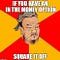# Just how bad is STT?

The rules of STT in options work this way:

Exercise of Options: Option exercise happens when you buy an option, do not square it off and it makes money on expiry. In this case, STT is charged on the underlying NIFTY price — which is around 10500 for NIFTY as of Dec 2017. The buyer of the option has to pay this STT.

Example: .125%*10500*75= 985 Rs, or 13 points on NIFTY.

Selling Options: When you sell an option, STT is charged on the premium, which is like in the order of 50–200 Rupees = .05%*200*75= 7.5 Rupees which is like 0.1 points on NIFTY. The seller pays this STT.

This also means when you square off an in-the-money option you have purchased instead of letting it expire, the STT is charged on the premium, as shown above. And that is significantly lower than STT on expiry.

As I am writing this, NIFTY Jan Future is at 10584. NIFTY 10600 Jan Call is worth 125 Rs. Let’s say NIFTY expired at 10700 in Jan and the option went in the money.

My profit without STT is 10700–10500–125 = 75 points, or 5625 Rs.

But my profit with STT is 10700–10500–125–13=62 points, or 4650 Rs.

That’s 17% lower!

# STT and Spreads

As I am writing this

NIFTY Dec FUT = 10550

NIFTY Dec 10550 Call = 30 Rs

NIFTY Dec 10600 Call = 10 Rs

10550–10600 Spread Cost = 30–10=20 Rs

Without STT:

Max Profit on a 10550–10600 Call Spread is 10600–10550–20 = 30 Rs

Max Loss on the spread is 20 Rs Premium

Reward/ Risk = 30/20=1.5

With STT:

Max Profit on a 10550–10600 Call Spread is 10600–10550–20- 13, which is the STT on 10550 Call if it goes in the money= 17 Rs

Max Loss on the spread is 20 Rs Premium

Reward/ Risk = 17/20=0.85

It makes buying spreads unattractive. Of course, if the expiry happens around 10600–10613, you can get lucky as you will get as much as 13 extra points on the 10550 Call you bought. Guess why? :)

2. Selling Spreads

NIFTY Dec FUT = 10550

NIFTY Dec 10550 Call = 30 Rs

NIFTY Dec 10600 Call = 10 Rs

Selling a 10550–10600 Spread gives = 30–10=20 Rs

Without STT:

Max Profit on a 10550–10600 Call Spread is the premium= 20 Rs

Max Loss on the spread is 10550–10600+20= -30

Reward/ Risk = 20/30 = 0.66

With STT:

Max Profit on a 10550–10600 Call Spread is the premium= 20 Rs

Max Loss on the spread is 10550–10600+20–13, which is the STT on 10600 call you bought = -43

Reward/ Risk = 20/43~0.5

It makes buying spreads unattractive. Of course, if the expiry happens around 10550–10563, you can get lucky as you will get as much as 13 extra points on the 10550 Call you sold. Guess why? :)

The answer to that lies here in a video tutorial about STT and here in a post about STT trap.

Takeaway

As Confucius said,

If you have an in-the-money option, always square it off, rather than letting it expire.Courtesy: Allthefakequotesevermadeup.com

How do you avoid STT? When do you start squaring off your positions? Help your fellow traders if you have some ideas to not pay STT. Let us know in the comments!

Options Trading For All

## More from Sensibull

Options Trading For All